# Accruals ratio

The accruals ratio is used by analysts to analyze the persistence and sustainability of a firm’s earnings. The closer the earnings are to operating cash flows, the higher the quality of the earnings. The main reason why earnings can be more easily manipulated than cash flows, is the fact that earnings are subject to accruals accounting.

To remove this bias, analysts will disaggregate earnings into cash flows and accruals. There are two methods we can use. The first is the balance sheet approach. This allows us to calculate the balance sheet accrual ratio. The second approach is the cash flow statement approach and this approach yields the cash flow based accruals ratio. The lower either ratio, the higher the earnings quality

On this page, we discuss both the balance sheet accrual formula and the cash flow based accrual ratio formula. We also implement an Excel example which can be downloaded at the bottom of this page.

## Balance sheet accrual ratio

Let’s start with the balance sheet approach. In this case, we can measure accruals as the change in net operating assets over a certain period.

• Net operating assets (NOA) is the difference between operating assets and operating liabilities
• Operating assets are total assets minus cash and marketable securities
• Operating liabilities are total liabilities minus total debt

Using a formula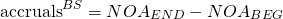Often, accruals are scaled such that they can can be compared across companies and over time. In that case, accruals are divided by the average NOA over the period. The resulting ratio is the balance sheet accrual ratio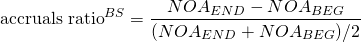## Cash flow based accruals ratio

The second way we can calculate accruals is using the cash flow approach. Under this approach, we take reported earnings and subtract cash flow from operating activities (CFO) and cash flow from investing (CFI) activities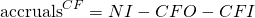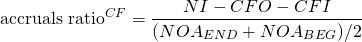## Accruals ratio example

Let’s finish with an example. The following table implements the approach using a numerical example. The following table implements both the balance sheet approach and the cash flow approach. The spreadsheet is available at the end of the page.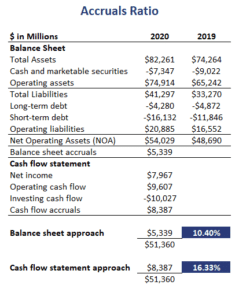## Summary

We discussed the two main methods to calculate the accrual ratio. This method is very popular when analyzing earnings quality. Another measure that can be calculate from the accruals calculated above is the accrued expenses turnover ratio.